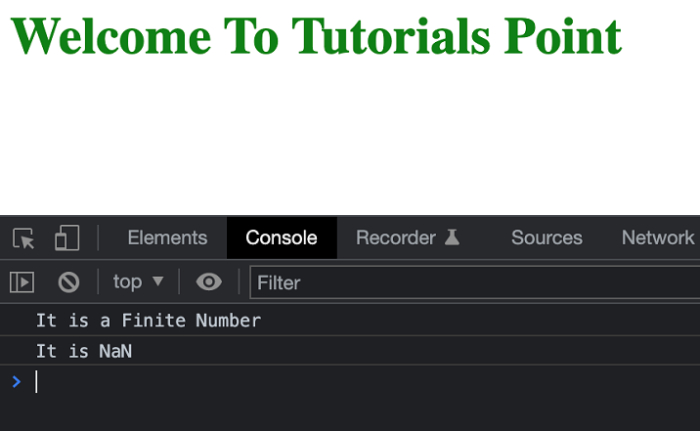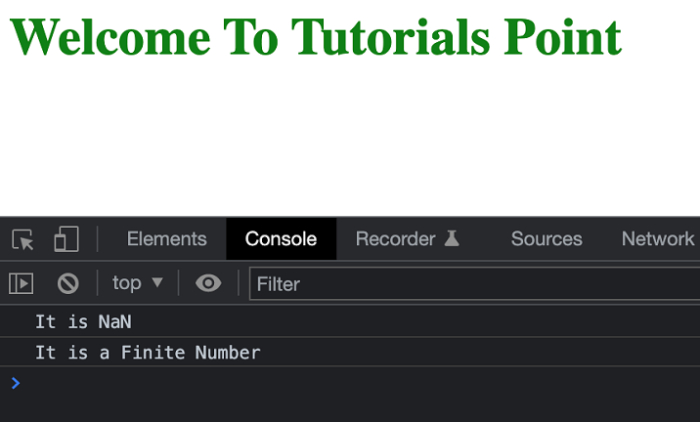# JavaScript: How to check if a number is NaN or finite?

Often we need to check whether a finite number or undefined returns the same to the user. In this article also we will be checking if a number is actually a finite number or Not a Number, i.e., NaN.

Below is the method to check whether a number is finite or not.

## isNaN()

This is a method provided by JavaScript to check whether a number is a finite number or not. If this method returns false, then the number is a finite number else the number is not finite.

## Syntax

isNaN(number )

## Example 1

In the below example, we are going to use the above method to check if the number is finite or not. The above method will either return true or false and based upon that we can decide if the number is finite or not.

# index.html

<!DOCTYPE html>
<html>
<title>Checking If a Number is Even</title>
<body>
<h1 style="color: green;">
Welcome To Tutorials Point
</h1>
<script>
function isFinite(x) {
if(isNaN(x)) {
return 'It is NaN';
} else {
return 'It is a Finite Number';
}
}
console.log(isFinite(21));
console.log(isFinite('Tutorials Point'));
</script>
</body>
</html>

## Output

You will get output in the console similar to the below screenshotisFinite −## isFinite()

Similar to isNaN(), we have a isFinite() method that basically checks whether a number is finite or not. If the number is finite it will return true else false.

## Syntax

isFinite(number )

## Example 2

In the below example, we are checking if the number is a finite number or not.

# index.html

<!DOCTYPE html>
<html>
<title>Checking If a Number is Even</title>
<body>
<h1 style="color: green;">
Welcome To Tutorials Point
</h1>
<script>
function isFinite(x) {
if(isNaN(x)) {
return 'It is NaN';
} else {
return 'It is a Finite Number';
}
}
console.log(isFinite('2C'));
console.log(isFinite(01010101));
</script>
</body>
</html>

## Output

You will get output in the console similar to the below screenshot −